EngliSea > M > math > 50 Trigonometry

A branch of mathematics that studies relationships between side lengths and angles of triangles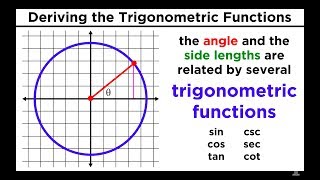02 Trigonometric Functions
Sine, Cosine, Tangent, Cosecant, Secant, and Cotangent
5021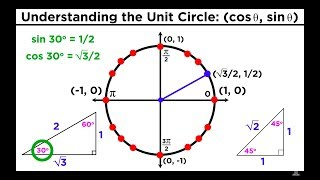03 The Easiest Way to Memorize the Trigonometric Unit Circle
The Easiest Way to Memorize the Trigonometric Unit Circle
5022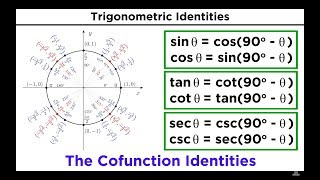04 Basic Trigonometric Identities
Basic Trigonometric Identities: Pythagorean Identities and Cofunction Identities
5107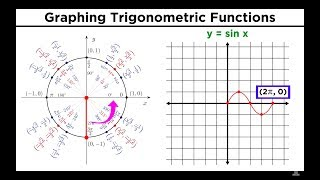05 Graphing Trigonometric Functions
Graphing Trigonometric Functions
5108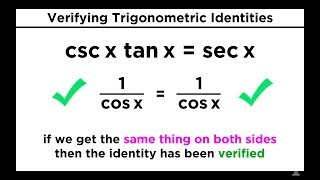07 Verifying Trigonometric Identities
Verifying Trigonometric Identities
5110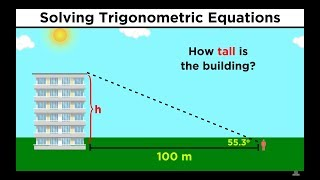09 Solving Trigonometric Equations
Solving Trigonometric Equations
5112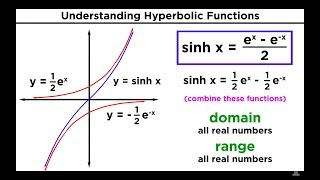Hyperbolic Functions: Definitions, Identities, Derivatives, and Inverses
Hyperbolic Functions: Definitions, Identities, Derivatives, and Inverses
3672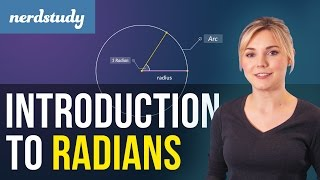Intro to Radians
right off the bat #math
3463

Pythagorean Identities
↑φ / →φ = ↗φ (↑φ)² + (→φ)² = 1 1 + (↙φ)² = (↓φ)² (↗φ)² + 1 = (←φ)²
9674-